# Multiplication

(diff) ← Older revision | Latest revision (diff) | Newer revision → (diff)

of numbers

One of the basic arithmetic operations. Multiplication consists in assigning to two numbers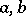(called the factors) a third number(called the product). Multiplication is denoted by the sign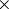or; in notations using letters the sign is, as a rule, omitted.

Multiplication of positive integers is defined in the following way by means of addition: the product of a numberby a numberis the numberequal to the sum ofsummands each of which is equal to; thus,The numberis called the multiplicand andthe multiplier. Multiplication of two positive rational numbers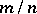and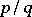is defined by the equation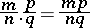(cf. Fraction). The product of two negative fractions is positive, and that of a positive and a negative fraction is negative, where the modulus of the product in both cases is equal to the product of the moduli of the factors. The product of irrational numbers is defined as the limit of the products of rational approximations of them. Multiplication of two complex numbers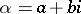and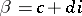is given by the formula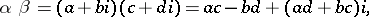or, in trigonometric form, by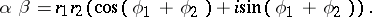Multiplication of numbers is commutative, associative and distributive on the left and right relative to addition (cf. Commutativity; Associativity; Distributivity). Further,,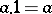.

In general algebra, multiplication may be any algebraic operation (-ary,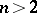); most frequently a binary operation (cf. Groupoid). In certain cases this operation is a generalization of the usual multiplication of numbers. E.g. multiplication of quaternions, multiplication of matrices and multiplication of transformations. However, properties of the multiplication of numbers (e.g. commutativity) may be lost in these cases.

How to Cite This Entry:
Multiplication. Encyclopedia of Mathematics. URL: http://encyclopediaofmath.org/index.php?title=Multiplication&oldid=13209
This article was adapted from an original article by O.A. Ivanova (originator), which appeared in Encyclopedia of Mathematics - ISBN 1402006098. See original article